## Pressure and buoyancy

11-12-99

Sections 10.1 - 10.6

### What is a fluid?

You probably think of a fluid as a liquid, but a fluid is simply anything that can flow. This includes liquids, but gases are fluids too.

### Mass density

When we talk about density it's usually mass density we're referring to. The mass density of an object is simply its mass divided by its volume. The symbol for density is the Greek letter rho, r :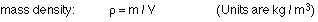Density depends on a few basic things. On a microscopic level, the density of an object depends on the weight of the individual atoms and molecules making up the object, and how much space there is between them. On a large-scale level, density depends on whether the object is solid, hollow, or something in between.

In general, liquids and solids have similar densities, which are of the order of 1000 kg / m3. Water at 4° C has a density of exactly this value; very dense materials like lead and gold have densities which are 10 - 20 times larger. Gases, on the other hand, have densities around 1 kg / m3, or about 1/1000 as much as water.

Densities are often given in terms of specific gravity. The specific gravity of an object or a material is the ratio of its density to the density of water at 4° C (this temperature is used because this is the temperature at which water is most dense). Gold has a specific gravity of 19.3, aluminum 2.7, and mercury 13.6. Note that these values are at standard temperature and pressure; objects will change size, and therefore density, in response to a change in temperature or pressure.

### Pressure

Density depends on pressure, but what exactly is pressure? Pressure is simply the force experienced by an object divided by the area of the surface on which the force acts. Note that the force here is the force acting perpendicular to the surface.

Pressure : P = F / A (The force is applied perpendicular to the area A)

The unit for pressure is the pascal, Pa. Pressure is often measured in other units (atmospheres, pounds per square inch, millibars, etc.), but the pascal is the unit that goes with the MKS (meter-kilogram-second) system.

When we talk about atmospheric pressure, we're talking about the pressure exerted by the weight of the air above us. The air goes up a long way, so even though it has a low density it still exerts a lot of pressure: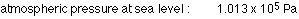On every square meter at the Earth's surface, then, the atmosphere exerts about 1.0 x 105 N of force. This is very large, but it is not usually noticed because there is generally air both inside and outside of things, so the forces applied by the atmosphere on each side of an object balance. It is when there are differences in pressure on two sides that atmospheric pressure becomes important. A good example is when you drink using a straw: you reduce the pressure at the top of the straw, and the atmosphere pushes the liquid up the straw and into your mouth.

### Pressure vs. depth in a static fluid

The pressure at any point in a static fluid depends only on the pressure at the top of the fluid and the depth of the point in the fluid. If point 2 lies a vertical distance h below point 1, there is a higher pressure at point 2; the pressure at the two points is related by the equation: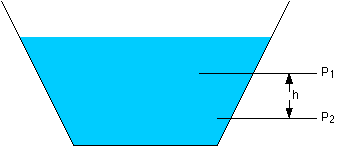Note that point 2 does not have to be directly below point 1; it is simply a vertical distance below point 1. This means that every point at a particular depth in a static fluid is at the same pressure.

### Pascal's principle

Pascal's principle can be used to explain how hydraulic systems work. A common example of such a system is the lift used to raise a car off the ground so it can be repaired at a garage.

Pascal's principle : Pressure applied to an enclosed fluid is transmitted undiminished to every part of the fluid, as well as to the walls of the container.

In a hydraulic lift, a small force applied to a small-area piston is transformed to a large force at a large-area piston. If a car sits on top of the large piston, it can be lifted by applying a relatively small force, the ratio of the forces being equal to the ratio of the areas of the pistons.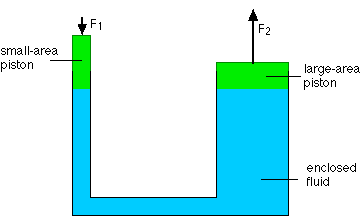Even though the force can be much less, the work done is the same. Work is force times the distance, so if the force on the large piston is 10 times larger than the force on the smaller piston, the distance it travels is 10 times smaller.

### Measuring pressure

The relationship between pressure and depth is often exploited in instruments that measure pressure. Two pressure gauges based on this principle are the closed-tube manometer and the open-tube manometer, which measure pressure by comparing the pressure at one end of the tube with a known pressure at the other end.

A standard mercury barometer is a closed-tube manometer, with one end sealed. The sealed end is close to zero pressure, while the other end is open to the atmosphere, or is connected to where the pressure is being measured. Because there is a pressure difference between the two ends of the tube, a column of fluid can be maintained in the tube, with the height of the column proportional to the pressure difference. If the closed end is at zero pressure, then the height of the column is proportional to the pressure at the other end.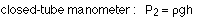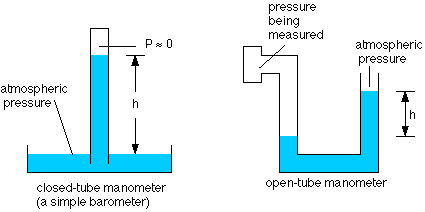In an open-tube manometer, one end of the tube is open to the atmosphere, and is thus at atmospheric pressure. The other end is connected to a region where the pressure is to be measured. Again, if there is a difference in pressure between the two ends of the tube, a column of fluid can be supported in the tube, with the height of the column being proportional to the pressure difference.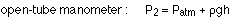The actual pressure, P2, is known as the absolute pressure; the pressure difference between the absolute pressure and atmospheric pressure is called the gauge pressure. Many pressure gauges give only the gauge pressure.

### Eureka!

According to legend, this is what Archimedes' cried when he discovered an important fact about buoyancy, so important that we call it Archimedes' principle (and so important that Archimedes allegedly jumped from his bath and ran naked through the streets after figuring it out).

Archimedes principle : An object that is partly or completely submerged in a fluid will experience a buoyant force equal to the weight of the fluid the object displaces.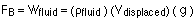The buoyant force applied by the fluid on the object is directed up. The force comes from the difference in pressure exerted on the top and bottom of an object. For a floating object, the top surface is at atmospheric pressure, while the bottom surface is at a higher pressure because it is in contact with the fluid at a particular depth in the fluid, and pressure increases with depth. For a completely-submerged object, the top surface is no longer at atmospheric pressure, but the bottom surface is still at a higher pressure because it's deeper in the fluid. In both cases, the difference in pressure results in a net upward force (the buoyant force) on the object.

### Example

A basketball floats in a bathtub of water. The ball has a mass of 0.5 kg and a diameter of 22 cm.

(a) What is the buoyant force?
(b) What is the volume of water displaced by the ball?
(c) What is the average density of the basketball?

(a) To find the buoyant force, simply draw a free-body diagram. The ball floats on the water, so there is no net force: the weight is balanced by the buoyant force, so:(b) By Archimedes' principle, the buoyant force is equal to the weight of fluid displaced. The weight is the mass times g, and the mass is the density times the volume, so:and then the volume displaced is simply:(c) To find the density of the ball, we need to determine the volume. This is given by:The density is then just the mass divided by this volume, so:Another way to find density is to use the volume of displaced fluid. For a floating object, the weight of the object equals the buoyant force, which equals the weight of the displaced fluid. Canceling out a factor of g gives: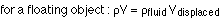So the density is: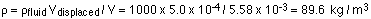The basketball is much less dense than water because it is filled with air. An object (or a fluid) will float on a fluid if its density is less than that of the fluid; if its density is larger than the fluid's, it will sink.